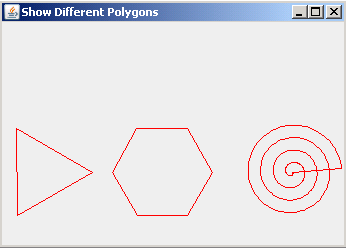Tutorials

# Draw Polygon in Graphics

A polygon is a closed path or circuit which is made by joining line segments. In polygon, each line segment intersects exactly two others line segments.

A polygon is a closed path or circuit which is made by joining line segments. In polygon, each line segment intersects exactly two others line segments.

# Draw Polygon in Graphics

In this section, you will learn how to draw a polygon in Graphics.

A polygon is a closed path or circuit which is made by joining line segments. In polygon, each line segment intersects exactly two others line segments. To draw a polygon, we have used the Polygon class.

Following code draws the polygon of three sides:

 for (int i = 0; i < 3; i++){ polygon1.addPoint((int) (40 + 50 * Math.cos(i * 2 * Math.PI / 3)), (int) (150 + 50 * Math.sin(i * 2 * Math.PI / 3)));} g.drawPolygon(polygon1);

Following code draws the polygon of six sides:

 for (int i = 0; i < 6; i++){ polygon2.addPoint((int) (160 + 50 * Math.cos(i * 2 * Math.PI / 6)), (int) (150 + 50 * Math.sin(i * 2 * Math.PI / 6)));} g.drawPolygon(polygon2);

Following code draws the spiral polygon:

 for (int i = 0; i < 360; i++) { double value = i / 360.0; polygon3.addPoint((int) (290 + 50 * value * Math.cos(8 * value * Math.PI)), (int) (150 + 50 * value * Math.sin(8 * value * Math.PI)));} g.drawPolygon(polygon3);}

The method addPoint() adds the coordinates to the polygon. The method drawPolygon() draws the polygon. The method setColor() sets the color to paint the polygon.

Here is the code of DrawPolygon.java

 import java.awt.*; import javax.swing.*; import java.awt.event.*; public class DrawPolygon extends JPanel {   public void paintComponent(Graphics g) {   super.paintComponent(g);   g.setColor(Color.red);  Polygon polygon1= new Polygon();  for (int i = 0; i < 3; i++){   polygon1.addPoint((int) (40 + 50 * Math.cos(i * 2 * Math.PI / 3)),   (int) (150 + 50 * Math.sin(i * 2 * Math.PI / 3)));  }  g.drawPolygon(polygon1);  Polygon polygon2= new Polygon();  for (int i = 0; i < 6; i++){   polygon2.addPoint((int) (160 + 50 * Math.cos(i * 2 * Math.PI / 6)),   (int) (150 + 50 * Math.sin(i * 2 * Math.PI / 6)));  }  g.drawPolygon(polygon2);   Polygon polygon3 = new Polygon();    for (int i = 0; i < 360; i++) {   double value = i / 360.0;   polygon3.addPoint((int) (290 + 50 * value * Math.cos(8 * value * Math.PI)),   (int) (150 + 50 * value * Math.sin(8 * value * Math.PI)));  }   g.drawPolygon(polygon3);  }     public static void main(String[] args) {   JFrame frame = new JFrame();   frame.setTitle("Show Different Polygons");   frame.setSize(350, 250);   Container contentPane = frame.getContentPane();   contentPane.add(new DrawPolygon());   frame.show();   } }

Output will be displayed as: